Search by Topic

Resources tagged with Enlargements and scale factors similar to Wedge on Wedge:

Filter by: Content type:
Age range:
Challenge level:

There are 16 results

Broad Topics > Transformations and constructions > Enlargements and scale factorsSquirty

Age 14 to 16 Challenge Level:

Using a ruler, pencil and compasses only, it is possible to construct a square inside any triangle so that all four vertices touch the sides of the triangle.Conical Bottle

Age 14 to 16 Challenge Level:

A right circular cone is filled with liquid to a depth of half its vertical height. The cone is inverted. How high up the vertical height of the cone will the liquid rise?Fit for Photocopying

Age 14 to 16 Challenge Level:

Explore the relationships between different paper sizes.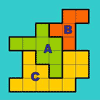The Rescaled Map

Age 14 to 16 Challenge Level:

We use statistics to give ourselves an informed view on a subject of interest. This problem explores how to scale countries on a map to represent characteristics other than land area.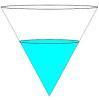Fill Me up Too

Age 14 to 16 Challenge Level:

In Fill Me Up we invited you to sketch graphs as vessels are filled with water. Can you work out the equations of the graphs?Matter of Scale

Age 14 to 16 Challenge Level:

Prove Pythagoras' Theorem using enlargements and scale factors.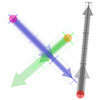Arrow Arithmetic 3

Age 14 to 16 Challenge Level:

How can you use twizzles to multiply and divide?Arrow Arithmetic 2

Age 14 to 16 Challenge Level:

Introduces the idea of a twizzle to represent number and asks how one can use this representation to add and subtract geometrically.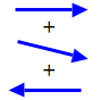Arrow Arithmetic 1

Age 14 to 16 Challenge Level:

The first part of an investigation into how to represent numbers using geometric transformations that ultimately leads us to discover numbers not on the number line.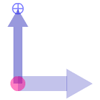Twizzle Arithmetic

Age 14 to 16 Challenge Level:

Arrow arithmetic, but with a twist.Hex

Age 11 to 14 Challenge Level:

Explain how the thirteen pieces making up the regular hexagon shown in the diagram can be re-assembled to form three smaller regular hexagons congruent to each other.Similar Rectangles

Age 14 to 16 Challenge Level:

Can you find the missing length?Who Is the Fairest of Them All ?

Age 11 to 14 Challenge Level:

Explore the effect of combining enlargements.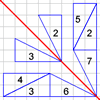Transformation Game

Age 11 to 14 Challenge Level:

Why not challenge a friend to play this transformation game?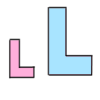Growing Rectangles

Age 11 to 14 Challenge Level:

What happens to the area and volume of 2D and 3D shapes when you enlarge them?L-triominoes

Age 14 to 16 Challenge Level:

L triominoes can fit together to make larger versions of themselves. Is every size possible to make in this way?